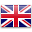English

# [LEARN in a minute] Video Surveillance Storage Calculation

## Background Information

How to calculate video storage capacity in one minute

I don’t know if any of you have experienced the same problem that I have. In the face of some customers or units that need video storage, hardware equipment, the number of equipment, monitoring points, and so on have been determined, but how to calculate the storage, how to calculate the storage capacity for customers? This article will help you solve this problem.

## Conceptual Framework

Tip: Understand the basics to get a better understanding of computing.

### What’s the codestream?

I’m sure you’ve all heard of the term, master stream, child stream. The primary stream is generally used for storage and the substream is used for network transmission. Colloquially, the main code stream is stored locally and the sub-code stream is suitable for image transmission over low bandwidth networks. Bitstream refers to the amount of data used in a video file per unit of time, also known as bit rate. The unit is Mbps.

### What is Mbps?

Mbps refers to the signal transmission rate, also known as bandwidth. Mbps = M bit/s.

### What is Bit?

The bit is the smallest unit of information representation, we usually say the hard disk capacity “GB”, inside the “B” which is Byte, is the basic unit of data storage, the two are completely different. `1Byte = 8 Bit`.

### Conversion between units

Bps (Bytes per second), i.e. bytes per second, because one byte corresponds to eight bits, so 1Bps = 8 bps.
`1Mbps = 100 0000 bps = 125000Bps = 125kBps = 0.125MBps`
`1P=1024T,1T=1024G,1G=1024M,1M=1024k`

## Learn to calculate

Formula: Surveillance Camera Code Streams ÷ 8 × 24 × 3600 × Roads × Video Storage Days = Capacity (in M)

Let me give you an example:

What is the storage capacity required for a surveillance camera with a bitstream of 2 to record for 30 days?
Capacity = 2 ÷ 8 × 24 × 3600 × 1 × 30 = 648000M0.7T
Next, you are introduced to several common video display formats.
Under the conditions of using the H.264 encoding format –

720P is calculated using a 2 Mbps code stream, and the amount of data stored for 30 days is 2 ÷ 8 × 3600 × 24 ÷ 1024 ÷ 1024 × 30 ≈ 0.7 T.

1080P is calculated using a 4Mbps bitstream, and the amount of data stored for 30 days is 4 ÷ 8 × 3600 × 24 ÷ 1024 ÷ 1024 × 30 ≈ 1.3 T.

## Learn How We Served 100+ Global Device Brands with our Products & Get Free Sample!!!